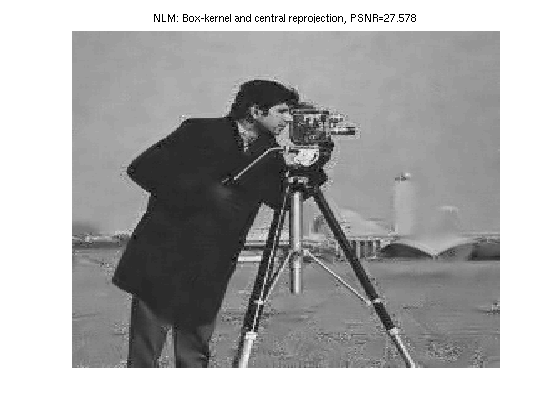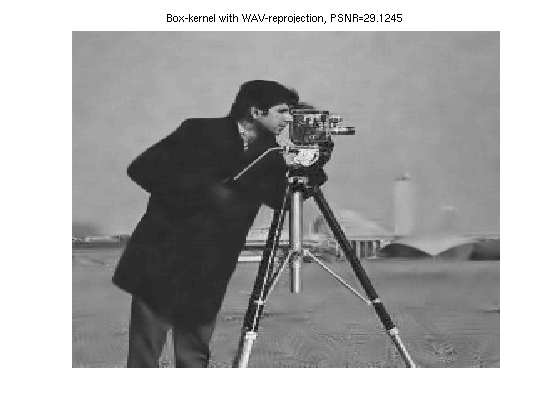# Reprojections for the NLM algorithm

## Visualization of several NLM reprojections: DEMO_reprojections.m

This script provides an overview of the results obtained by various reprojections for the NLM algorithm. We mainly use the box-kernel, and the Euclidean norm to compare patches. In this demo we present the results for two images corrupted by Gaussian noise with known variance $\sigma^2$. We emphasize that the reprojection based on the inverse variance weights leads to the best results. NLM variants for the Cameraman image with $\sigma=20$ with searching zone of size $9\times 9$ and patches of size $9\times 9$. See also README.TXT in the associate zip file for more details.

Authors: $\textbf{J. Salmon}$ and $\textbf{Y. Strozecki}$

The algorithm is coded in C and a mexisation step is needed for Matlab.

%mex C_code/Nlmeans_flat.c;
%mex C_code/Nlmeans_flat_reprojection_mini.c;
%mex C_code/Nlmeans_flat_reprojection_uae.c;
%mex C_code/Nlmeans_subsampling_matrice_variance_double.c;
%mex C_code/Nlmeans_original.c;


## Images genereration: original and noisy one.

tic
sigma=20;
[I,IB1]=genere_figure_bruit(4,sigma);
titre=strcat('Noisy: PSNR=',num2str(psnr(IB1,I)));
plot_perso(IB1,titre)## Box-kernel and central reprojection

r1=4; % half width of the searching zone
w1=4; % half width of the patch
threshold=1/(2*w1+1)^2*qchisq(0.99,(2*w1+1)^2)*(2*sigma^2);
R2=Nlmeans_flat(IB1,w1,r1,threshold,2);
titre=strcat('NLM: Box-kernel and central reprojection, PSNR=',...
num2str(psnr(R2,I)));
plot_perso(R2,titre)## Gaussian kernel with central reprojection

h=1*sigma^2;
w1=4;
threshold=1000000;
bord=2; %% 1: toric, 2:symmetric world
R2=Nlmeans_original(IB1,w1,r1,1/h,threshold,bord);
titre=strcat('NLM: Gaussian kernel with central reprojection', ...
'PSNR=',num2str(psnr(R2,I)));
plot_perso(R2,titre)## Box kernel with uniform-reprojection

w1=9;
threshold=1/(w1)^2*qchisq(0.99,(w1)^2)*(2*sigma^2);
R2=Nlmeans_flat_reprojection_uae(IB1,w1,r1,threshold);
titre=strcat('NLM: Box-kernel with uniform reprojecction, PSNR=',...
num2str(psnr(R2,I)));
plot_perso(R2,titre)## Box-kernel with minimizing variance reprojection

threshold=1/(w1)^2*qchisq(0.99,(w1)^2)*(2*sigma^2);
R2=Nlmeans_flat_reprojection_mini(IB1,w1,r1,threshold);
titre=strcat('Box-kernel minimizing variance reprojection, PSNR=',...
num2str(psnr(R2,I)));
plot_perso(R2,titre)## Box-kernel with weigthed averaging reprojection based on the inverse variance (WAV)

threshold=1/(w1)^2*qchisq(0.99,(w1)^2)*(2*sigma^2);
R2=Nlmeans_subsampling_matrice_variance_double(IB1,...
IB1,w1,r1,threshold,1);
titre=strcat('Box-kernel with WAV-reprojection, PSNR=',...
num2str(psnr(R2,I)));
plot_perso(R2,titre)## Wav-reprojection with two size of patches

w_big=9;
w_small=2;
threshold1=1/(w_big)^2*qchisq(0.99,(w_big)^2)*(2*sigma^2);
threshold2=1/(w_small)^2*qchisq(0.75,(w_small)^2)*2*sigma^2;
[nl1,normalisation1]=Nlmeans_subsampling_matrice_variance_double(...
IB1,IB1,w_big,r1,threshold1,1);
[nl2,normalisation2]=Nlmeans_subsampling_matrice_variance_double(...
IB1,IB1,w_small,r1,threshold2,1);
R2=(normalisation1/w_big.*nl1+nl2.*normalisation2/w_small)./...
(normalisation1/w_big+normalisation2/w_small);
titre=strcat(strcat('Box-kernel combining two sizes of '...
,' patches with ',' WAV reprojection, PSNR=',...
num2str(psnr(R2,I))));
plot_perso(R2,titre)

disp(['Demo completed in ' num2str(toc) ' seconds']);

Demo completed in 13.6733 secondsPapers:

"From Patches to Pixels in Non-Local methods: Weighted-Average Reprojection"
J. Salmon and Y. , ICIP, 2010, PDF.

"Patch Reprojections for Non Local Methods"
J. Salmon and Y. , Signal Processing, 2011, PDF.

Corresponding Matlab toolbox ZIP.